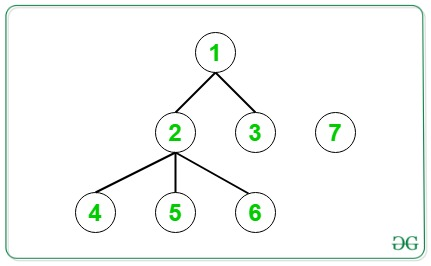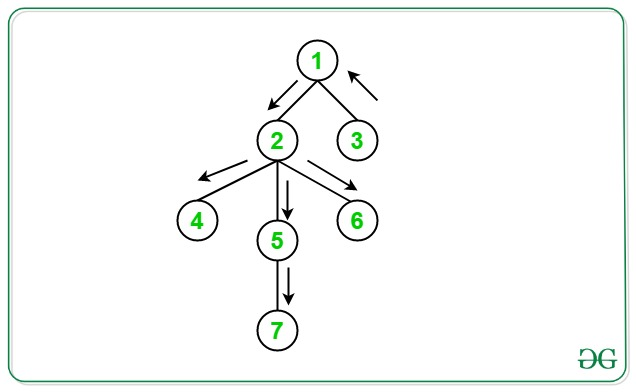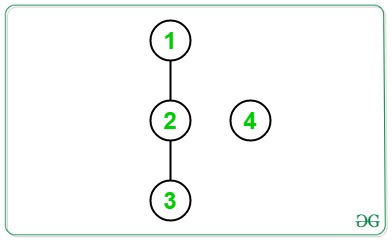Open in App
Not now

# Diameters for each node of Tree after connecting it with given disconnected component

• Last Updated : 22 Jun, 2021

Given a Tree having N nodes connected by N − 1 edge and a single disconnected node, the task is to find the diameters for each node of the given Tree after connecting it with the given disconnected component.

Example:

Input:Output: 3 3 4 4 4 4
Explanation:
Initially diameter of tree = 3
If an edge is added between node 1 and node 7, then the diameter of the tree is equal to 3(7 -> 1 -> 2 -> 5).
If an edge is added between node 2 and node 7, then the diameter of the tree is equal to 3(3 -> 1 -> 2 -> 5).
If an edge is added between node 3 and node 7, then the diameter of the tree is equal to 4(7 -> 3 -> 1 -> 2 -> 5).
If an edge is added between node 4 and node 7, then the diameter of the new tree is equal to 4(7-> 4 -> 2 -> 1 -> 3).
If an edge is added between node 5 and node 7, then the diameter of the new tree will be 4(7-> 5 -> 2 -> 1 -> 3).
If an edge is added between node 6 and node 7, then the diameter of the new tree will be 4((7-> 6 -> 2 -> 1 -> 3)).Input:Output: 3 2 3
Explanation:
Initial diameter of the tree = 2
If an edge is added between node 1 and node 4, then the diameter of the tree is equal to 3(4 -> 1 -> 2 -> 3).
If an edge is added between node 2 and node 4, then the diameter of the tree is equal to 2(4 -> 2 -> 3).
If an edge is added between node 3 and node 4, then the diameter of the tree is equal to 3(4 -> 3 -> 2 -> 1).

Approach: To solve the problem, the following observations need to be made:

• The diameter increases by 1 when the disconnected node is connected to an edge which forms the end of the diameter.
• For all other nodes, the diameter remains unchanged on connecting the disconnected node.

Follow the steps given below to solve the problem based on the above observations:

1. Perform the DFS traversal of the given tree.
2. While traversing, keep track of the farthest distance traveled and the farthest node.
3. Now, perform DFS from the farthest node obtained from the above step and keep track of the farthest node from this node.
4. Now, perform DFS and keep adding nodes in a Map that is farthest from the two nodes obtained from the above steps separately.

Below is the implementation of the above approach:

## C++

 `// C++ Program to implement``// the above approach``#include ``using` `namespace` `std;` `// Keeps track of the farthest``// end of the diameter``int` `X = 1;` `// Keeps track of the length of``// the diameter``int` `diameter = 0;` `// Stores the nodes which are at``// ends of the diameter``map<``int``, ``bool``> mp;` `// Perform DFS on the given tree``void` `dfs(``int` `current_node, ``int` `prev_node,``         ``int` `len, ``bool` `add_to_map,``         ``vector >& adj)``{``    ``// Update diameter and X``    ``if` `(len > diameter) {``        ``diameter = len;``        ``X = current_node;``    ``}` `    ``// If current node is an end of diameter``    ``if` `(add_to_map && len == diameter) {``        ``mp[current_node] = 1;``    ``}` `    ``// Traverse its neighbors``    ``for` `(``auto``& it : adj[current_node]) {``        ``if` `(it != prev_node)``            ``dfs(it, current_node, len + 1,``                ``add_to_map, adj);``    ``}``}` `// Function to call DFS for the``// required purposes``void` `dfsUtility(vector >& adj)``{``    ``// DFS from a random node and find``    ``// the node farthest from it``    ``dfs(1, -1, 0, 0, adj);` `    ``int` `farthest_node = X;` `    ``// DFS from X to calculate diameter``    ``dfs(farthest_node, -1, 0, 0, adj);` `    ``// DFS from farthest_node to find``    ``// the farthest node(s) from it``    ``dfs(farthest_node, -1, 0, 1, adj);` `    ``// DFS from X (other end of diameter) and``    ``// check the farthest node(s) from it``    ``dfs(X, -1, 0, 1, adj);``}` `void` `printDiameters(vector >& adj)``{``    ``dfsUtility(adj);` `    ``for` `(``int` `i = 1; i <= 6; i++) {` `        ``// If node i is the end of``        ``// a diameter``        ``if` `(mp[i] == 1)` `            ``// Increase diameter by 1``            ``cout << diameter + 1 << ``",  "``;` `        ``// Otherwise``        ``else` `            ``// Remains unchanged``            ``cout << diameter << ``",  "``;``    ``}``}` `// Driver Code``int` `main()``{` `    ``/* constructed tree is``            ``1``           ``/ \``          ``2   3    7``         ``/|\``        ``/ | \``       ``4  5  6    */` `    ``vector > adj(7);` `    ``// creating undirected edges``    ``adj.push_back(2);``    ``adj.push_back(1);``    ``adj.push_back(3);``    ``adj.push_back(1);``    ``adj.push_back(4);``    ``adj.push_back(2);``    ``adj.push_back(5);``    ``adj.push_back(2);``    ``adj.push_back(6);``    ``adj.push_back(2);` `    ``printDiameters(adj);` `    ``return` `0;``}`

## Java

 `// Java Program to implement``// the above approach``import` `java.util.*;``class` `GFG{` `// Keeps track of the farthest``// end of the diameter``static` `int` `X = ``1``;` `// Keeps track of the length of``// the diameter``static` `int` `diameter = ``0``;` `// Stores the nodes which are at``// ends of the diameter``static` `HashMap mp = ``new` `HashMap<>();` `// Perform DFS on the given tree``static` `void` `dfs(``int` `current_node, ``int` `prev_node,``                 ``int` `len, ``boolean` `add_to_map,``                ``Vector []  adj)``{``    ``// Update diameter and X``    ``if` `(len > diameter)``    ``{``        ``diameter = len;``        ``X = current_node;``    ``}` `    ``// If current node is an end of diameter``    ``if` `(add_to_map && len == diameter)``    ``{``        ``mp.put(current_node, ``true``);``    ``}` `    ``// Traverse its neighbors``    ``for` `(``int` `it : adj[current_node])``    ``{``        ``if` `(it != prev_node)``            ``dfs(it, current_node, len + ``1``,``                ``add_to_map, adj);``    ``}``}` `// Function to call DFS for the``// required purposes``static` `void` `dfsUtility(Vector []  adj)``{``    ``// DFS from a random node and find``    ``// the node farthest from it``    ``dfs(``1``, -``1``, ``0``, ``false``, adj);` `    ``int` `farthest_node = X;` `    ``// DFS from X to calculate diameter``    ``dfs(farthest_node, -``1``, ``0``, ``false``, adj);` `    ``// DFS from farthest_node to find``    ``// the farthest node(s) from it``    ``dfs(farthest_node, -``1``, ``0``, ``true``, adj);` `    ``// DFS from X (other end of diameter) and``    ``// check the farthest node(s) from it``    ``dfs(X, -``1``, ``0``, ``true``, adj);``}` `static` `void` `printDiameters(Vector [] adj)``{``    ``dfsUtility(adj);` `    ``for` `(``int` `i = ``1``; i <= ``6``; i++)``    ``{` `        ``// If node i is the end of``        ``// a diameter``        ``if` `(mp.containsKey(i) &&``            ``mp.get(i) == ``true``)` `            ``// Increase diameter by 1``            ``System.out.print(diameter + ``1` `+ ``",  "``);` `        ``// Otherwise``        ``else` `            ``// Remains unchanged``            ``System.out.print(diameter + ``",  "``);``    ``}``}` `// Driver Code``public` `static` `void` `main(String[] args)``{` `    ``/* constructed tree is``            ``1``           ``/ \``          ``2   3    7``         ``/|\``        ``/ | \``       ``4  5  6    */` `    ``Vector []adj = ``new` `Vector[``7``];``    ``for` `(``int` `i = ``0``; i < adj.length; i++)``        ``adj[i] = ``new` `Vector();``  ` `    ``// creating undirected edges``    ``adj[``1``].add(``2``);``    ``adj[``2``].add(``1``);``    ``adj[``1``].add(``3``);``    ``adj[``3``].add(``1``);``    ``adj[``2``].add(``4``);``    ``adj[``4``].add(``2``);``    ``adj[``2``].add(``5``);``    ``adj[``5``].add(``2``);``    ``adj[``2``].add(``6``);``    ``adj[``6``].add(``2``);` `    ``printDiameters(adj);``}``}` `// This code is contributed by PrinciRaj1992`

## Python3

 `# Python3 program to implement``# the above approach``from` `collections ``import` `defaultdict` `# Keeps track of the farthest``# end of the diameter``X ``=` `1` `# Keeps track of the length of``# the diameter``diameter ``=` `0` `# Stores the nodes which are at``# ends of the diameter``mp ``=` `defaultdict(``lambda` `:``0``)` `# Perform DFS on the given tree``def` `dfs(current_node, prev_node,``        ``len``, add_to_map, adj):``            ` `    ``global` `diameter, X``    ` `    ``# Update diameter and X``    ``if` `len` `> diameter:``        ``diameter ``=` `len``        ``X ``=` `current_node``    ` `    ``# If current node is an end of diameter``    ``if` `add_to_map ``and` `len` `=``=` `diameter:``        ``mp[current_node] ``=` `1``    ` `    ``# Traverse its neighbors``    ``for` `it ``in` `adj[current_node]:``        ``if` `it !``=` `prev_node:``            ``dfs(it, current_node, ``len` `+` `1``,``                ``add_to_map, adj)``            ` `# Function to call DFS for the``# required purposes``def` `dfsUtility(adj):``    ` `    ``# DFS from a random node and find``    ``# the node farthest from it``    ``dfs(``1``, ``-``1``, ``0``, ``0``, adj)``    ` `    ``farthest_node ``=` `X``    ` `    ``# DFS from X to calculate diameter``    ``dfs(farthest_node, ``-``1``, ``0``, ``0``, adj)``    ` `    ``# DFS from farthest_node to find``    ``# the farthest node(s) from it``    ``dfs(farthest_node, ``-``1``, ``0``, ``1``, adj)``    ` `    ``# DFS from X (other end of diameter) and``    ``# check the farthest node(s) from it``    ``dfs(X, ``-``1``, ``0``, ``1``, adj)``    ` `def` `printDiameters(adj):``    ` `    ``global` `diameter``    ``dfsUtility(adj)``    ` `    ``for` `i ``in` `range``(``1``, ``6` `+` `1``):``        ` `        ``# If node i is the end of``        ``# a diameter``        ``if` `mp[i] ``=``=` `1``:``            ` `            ``# Increase diameter by 1``            ``print``(diameter ``+` `1``, end ``=` `", "``)``            ` `        ``# Otherwise``        ``else``:``            ` `            ``# Remains unchanged``            ``print``(diameter, end ``=` `", "``)``            ` `# Driver code``    ` `# constructed tree is``#         1``#        / \``#      2   3``#    / | \``#   4  5  6``#      |``#      7` `adj ``=` `[]``for` `i ``in` `range``(``7``):``    ``adj.append([])``    ` `# Creating undirected edges``adj[``1``].append(``2``)``adj[``2``].append(``1``)``adj[``1``].append(``3``)``adj[``3``].append(``1``)``adj[``2``].append(``4``)``adj[``4``].append(``2``)``adj[``2``].append(``5``)``adj[``5``].append(``2``)``adj[``2``].append(``6``)``adj[``6``].append(``2``)` `printDiameters(adj)` `# This code is contributed by Stuti Pathak`

## C#

 `// C# Program to implement``// the above approach``using` `System;``using` `System.Collections.Generic;``class` `GFG {` `    ``// Keeps track of the farthest``    ``// end of the diameter``    ``static` `int` `X = 1;` `    ``// Keeps track of the``    ``// length of the diameter``    ``static` `int` `diameter = 0;` `    ``// Stores the nodes which are at``    ``// ends of the diameter``    ``static` `Dictionary<``int``, Boolean> mp``        ``= ``new` `Dictionary<``int``, Boolean>();` `    ``// Perform DFS on the given tree``    ``static` `void` `dfs(``int` `current_node, ``int` `prev_node,``                    ``int` `len, ``bool` `add_to_map,``                    ``List<``int``>[] adj)``    ``{``        ``// Update diameter and X``        ``if` `(len > diameter)``        ``{``            ``diameter = len;``            ``X = current_node;``        ``}` `        ``// If current node is an end of diameter``        ``if` `(add_to_map && len == diameter)``        ``{``            ``mp.Add(current_node, ``true``);``        ``}` `        ``// Traverse its neighbors``        ``foreach``(``int` `it ``in` `adj[current_node])``        ``{``            ``if` `(it != prev_node)``                ``dfs(it, current_node, len + 1,``                    ``add_to_map, adj);``        ``}``    ``}` `    ``// Function to call DFS for``    ``// the required purposes``    ``static` `void` `dfsUtility(List<``int``>[] adj)``    ``{` `        ``// DFS from a random node and find``        ``// the node farthest from it``        ``dfs(1, -1, 0, ``false``, adj);` `        ``int` `farthest_node = X;` `        ``// DFS from X to calculate diameter``        ``dfs(farthest_node, -1, 0, ``false``, adj);` `        ``// DFS from farthest_node to find``        ``// the farthest node(s) from it``        ``dfs(farthest_node, -1, 0, ``true``, adj);` `        ``// DFS from X (other end of diameter) and``        ``// check the farthest node(s) from it``        ``dfs(X, -1, 0, ``true``, adj);``    ``}` `    ``static` `void` `printDiameters(List<``int``>[] adj)``    ``{``        ``dfsUtility(adj);` `        ``for` `(``int` `i = 1; i <= 6; i++)``        ``{` `            ``// If node i is the end``            ``// of a diameter``            ``if` `(mp.ContainsKey(i) && mp[i] == ``true``)` `                ``// Increase diameter by 1``                ``Console.Write(diameter + 1 + ``",  "``);` `            ``// Otherwise``            ``else` `                ``// Remains unchanged``                ``Console.Write(diameter + ``",  "``);``        ``}``    ``}` `    ``// Driver Code``    ``public` `static` `void` `Main(String[] args)``    ``{` `        ``/* constructed tree is``            ``1``           ``/ \``          ``2   3    7``         ``/|\``        ``/ | \``       ``4  5  6    */` `        ``List<``int``>[] adj = ``new` `List<``int``>[ 7 ];``        ``for` `(``int` `i = 0; i < adj.Length; i++)``            ``adj[i] = ``new` `List<``int``>();` `        ``// creating undirected edges``        ``adj.Add(2);``        ``adj.Add(1);``        ``adj.Add(3);``        ``adj.Add(1);``        ``adj.Add(4);``        ``adj.Add(2);``        ``adj.Add(5);``        ``adj.Add(2);``        ``adj.Add(6);``        ``adj.Add(2);``        ``printDiameters(adj);``    ``}``}` `// This code is contributed by Amit Katiyar`

## Javascript

 ``

Output:

`3,  3,  4,  4,  4,  4,`

Time Complexity: O(V + E), where V is the number of vertices and E is the number of edges in the graph.
Auxiliary Space: O(V)

My Personal Notes arrow_drop_up